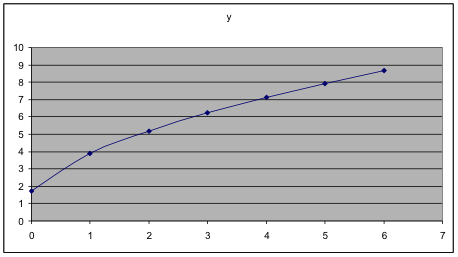## Section 4. Formulas and functions

We have similar issues about order of operations when we enter formulas into a spreadsheet as when we enter them into a calculator. In these two types of problems below, it is necessary to insert parentheses. When we write these by hand, the order of operations is implied by the placement of the expressions, so that when we type them in, we must use parentheses to compensate for that.

 Evaluate each expression In a spreadsheet, if the x-value is in cell A2 ${{3}^{2x}}$, where $x=3$ =3^(2*A2) $\frac{{{x}^{2}}-6x}{4x+2}$, where $x=3$ =(A2^2-6*A2)/(4*A2+2) $\sqrt{4x+9}$ =SQRT(4*A2+9)                  or                          =(4*A2+9)^0.5

In addition to the usual mathematical operations, there are various named functions you may use in spreadsheet formulas.

 Named function Example (usual notation) Example (spreadsheet notation) Square Root $\sqrt{4x-5}$ =SQRT(4*A2-5) Average (of a set of five numbers, in cells A2 through A6) $\frac{{{x}_{1}}+{{x}_{2}}+{{x}_{3}}+{{x}_{4}}+{{x}_{5}}}{5}$ =AVERAGE(A2:A6) Maximum (of a set of seven numbers, in B2 through B8) $\max({{x}_{1}},{{x}_{2}},{{x}_{3}},{{x}_{4}},{{x}_{5}},{{x}_{6}},{{x}_{7}})$ =MAX(B2:B8) Pi (the value 3.14…) $\pi$ =PI()

To find additional functions, in Excel, choose Insert > Function and explore the dialog box. Many of the functions will be things you don’t know about, but some will have familiar names. Explore them.

Example 13.   Use a spreadsheet to graph $y=\sqrt{12x+3}$ for input values between 0 and 6.

Solution:   Put in the values for x, then insert the formula. Then graph.

 A B 1 x y 2 0 1.732051 3 1 3.872983 4 2 5.196152 5 3 6.244998 6 4 7.141428 7 5 7.937254 8 6 8.660254

The formula in cell B2 is

=SQRT(12*A2+3)

Then that formula was copied to cells B3 through B8.Example 14. Put the formula for the value into the spreadsheet and see what number it gives.

Solution. In cell A1, put =PI(). Enter that. See that it gives the value 3.14 … with high precision, as does the key on a calculator.

Example 15. Use a spreadsheet to compute the average of these six test grades: 72, 85, 69, 79, 92, 71

Solution:

 A 1 72 2 85 3 69 4 79 5 92 6 71

Cell C4:

 78 average

First, type the scores into a blank spreadsheet. (In this case, copying and pasting doesn’t work because the scores above are not in separate cells of a table in the original docu­ment.)   If you put them in the upper left corner, they will be in cells A1 through A6.

Next choose another cell to put the formula in. If you choose cell C4, then in C4, put this formula =AVERAGE(A1:A6)

As soon as you enter that formula, the average of the six numbers, which is 78, will appear in the cell.

To “play” with this, go back to the cells with the data and replace the first value of 72 with 102. Notice that the average in cell C4 changes to 83.

Example 16. Use a spreadsheet to find the maximum of all the output data values in the dataset below.

Solution:

 A B C 1 Year Men Women 2 2000 67 62 3 2001 57 55 4 2002 63 59 5 2003 49 68 6 2004 52 42

Cell F3:

 68 maximum

First, copy and paste these data values into a blank spreadsheet.   If you paste the whole set into the upper left corner, the output data will be in cells B2 through C6

Next choose another cell to put the formula in. If you choose cell F3 then, in cell F3, put this formula     =MAX(B2:C6)

As soon as you enter this formula, that cell should show 68, which is the maximum of these numbers.

Now, to “play” with this, go back to the cells with the data and replace the first value in the “Men” column, 67, with the number 85. Notice that the maximum, over in cell F3, changes to the new maximum 85.

Note: well-organized data will have all the data in a single column (or sometimes a single row), except when there are subcategories like those shown here.